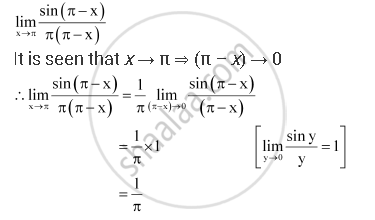Share

# Evaluate the Given Limit Lim_(X -> Pi) (Sin(Pi - X))/(Pi (Pi - X)) - Mathematics

#### Question

Evaluate the Given limit lim_(x -> pi) (sin(pi - x))/(pi (pi - x))

#### SolutionIs there an error in this question or solution?

#### APPEARS IN

NCERT Solution for Mathematics Textbook for Class 11 (2018 (Latest))
Chapter 13: Limits and Derivatives
Q: 15 | Page no. 302

#### Video TutorialsVIEW ALL 

Solution Evaluate the Given Limit Lim_(X -> Pi) (Sin(Pi - X))/(Pi (Pi - X)) Concept: Limits of Trigonometric Functions.
S